## Example Questions

### Example Question #1 : How To Find The Cosine Of An Angle

If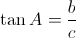, where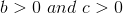and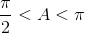,

then what is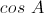?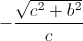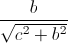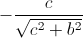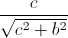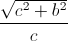Explanation:

In the triangle below, the tangent of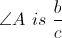, or the opposite side of the angle divided by the adjacent side of the angle. According to the Pythagorean

Theorem, the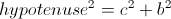Thus the hypotenuse equals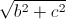.

The cosine of an angle is the adjacent side of the angle divided by the hypotenuse of the triangle, giving us.

However, since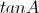is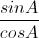, and when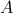is between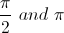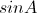is positive while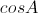is negative. Thus,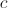is negative, giving us the final answer of.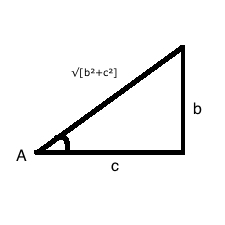### Example Question #1 : How To Find The Cosine Of An Angle

What is cos θ?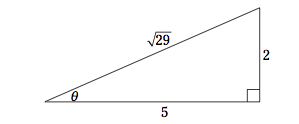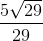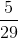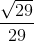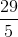Explanation:

cos = adjacent/hypotenuse =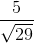To get the radical out of the denominator, we multiply: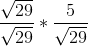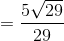### Example Question #3 : How To Find The Cosine Of An Angle

What is the cosine of the angle formed between the-axis and the line passing through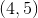with a slope of?  Round to the nearest hundredth.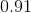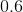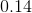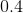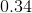Explanation:

You do not even need to compute the line for this question.  All that you need to do is note that you can make a little right triangle with a height ofand a base of, which you get from the slope of the line.  So, to compute the cosine, you will need to find the hypotenuse using the Pythagorean theorem: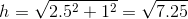So, our little triangle looks like: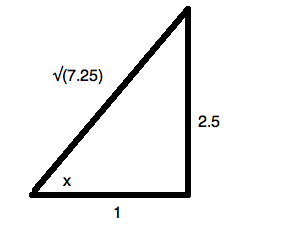The cosine will be the adjacent side,, divided by the hypotenuse,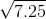: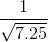, or approximately.

### Example Question #1 : How To Find The Cosine Of An Angle

What is the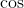of an angle with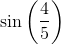?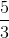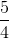Explanation:

Since the sine of the angle is, that means the opposite side of the triangle isand the hypotenuse is. Automatically, you know this is a special 3-4-5 right triangle, and that the missing side is 3. If not, you could also find the 3rd side by doing Pythagorean Theorem. This gives you your answer of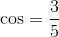### Example Question #5 : How To Find The Cosine Of An Angle

Right triangle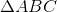has sides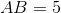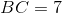and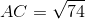. What is the cosine of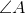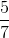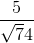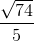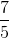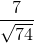Explanation:

SOHCAHTOA tells us that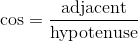, and we know the hypotenuse is the longest side of the triangle,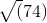. Our adjacent side will be the other side that hasas a vertex,.

Thus,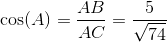.

### Example Question #6 : How To Find The Cosine Of An Angle

Right trianglehas sides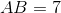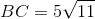and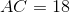. What is the cosine of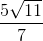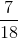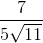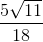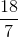SOHCAHTOA tells us that, and we know the hypotenuse is the longest side of the triangle,. Our adjacent side will be the other side that hasas a vertex,.
Thus,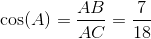.